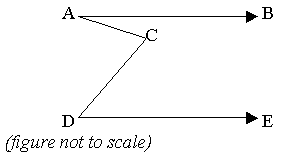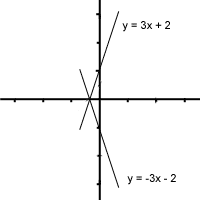50万+已关注

# SAT数学练习题整理10

2019-02-08 17:26:00来源：网络

新东方在线SAT频道为大家带来SAT数学练习题整理10一文，希望对大家SAT备考有所帮助。更多精彩尽请关注新东方在线SAT频道!

1. Courier charges for packages to a certain destination are 65 cents for the first 250 grams and 10 cents for each additional 100 grams or part thereof. What could be the weight in grams of a package for which the charge is \$1.55 ?

A. 1155

B. 1145

C. 1040

D. 950

E. 2592. AB and DE are parallel. Angle BAC = 30 , angle CDE = 50. What is the measure of angle ACD ?

A. 100

B. 90

C. 80

D. 70

E. cannot be determined from the information

3. If x / y is an integer, which of the following statements must be true?

A. both x and y are integers

B. x is an integer

C. either x or y is negative

D. y / x is an integer

E. x = ny where n is an integer

4. What is the average of four tenths and five thousandths?

A. 25002

B. 2502

C. 0.225

D. 0.2025

E. 0.02025

5. What is the simplified result of following the steps above in order?

(1) add 5y to 2x

(2) multiply the sum by 3

(3) subtract x + y from the product

A. 5x + 14y

B. 5x + 16y

C. 5x + 5y

D. 6x + 4y

E. 3x + 12y

6. If the equation of a line p in the coordinate plane is y = 3x + 2, what is the equation of line q which is a reflection of line p in the x-axis?

A. y = -3x + 2

B. y = -3x - 2

C. y = 3x - 2

D. y = -1/3x - 5

E. y = -1/3x + 5

7. If y ¤ x = y2x for all positive integers, then (3 ¤ 4) ¤ 2 =

A. 38

B. 312

C. 316

D. 324

E. 332

8. The first term in a sequence is 1 and the second term is 5. From the third term on each term is the average (arithmetic mean) of all preceding terms. What is the 25th term in the sequence?

A. 2.5

B. 3

C. 5

D. 25

E. 509. The solid brick shown is made of small bricks of side 1. When the large brick is disassembled into its component small bricks, the total surface area of all the small bricks is how much greater than the surface area of the large brick?

A. 32

B. 40

C. 60

D. 72

E. 80

10. (3x + 2) (2x - 5) = ax2 + kx + n .

What is the value of a - n + k ?

A. 5

B. 8

C. 9

D. 10

E. 11

Explanation:

The weight will be 250g plus (1.55 - 0.65)/0.10 units of 100g

250 + 900 = 1150

This is the maximum weight that can be sent at that price. But, weights exceeding

250 + 800 will also get charged this amount (that is what the ‘part thereof’ implies).

Hence a package weighing 1145 (ans. B) will be charged \$1.55

Explanation:

Imagine a third parallel line drawn through C. The angle ACD will be made of two angles, one alternate to BAC, and therefore = 30. The other is alternate to CDE and therefore = 50. Total for angle ACD = 80

Explanation:

You can eliminate answer choices by picking numbers.

In case (A), x and y could, for example, both be equal fractions and x/y would be an integer.

For the same reason (B) does not have to be correct.

There is no reason for either of them to be negative.

Answer (D) does not need to be correct. Consider x/y = 6/3. Then y/x = 3/6, which is a fraction not an integer.

But rearranging x/y = n, we get x = ny which is therefore always true.

Explanation:

Four tenths = 0.4

Five thousandths = 0.005

The average is (0.4 + 0.005)/2 = 0.2025

Explanation:

Step one 2x + 5y

Step two 3(2x + 5y) = 6x + 15y

Step three (6x + 15y) - (x +y) = 6x + 15y - x - y (watch that last minus sign!)

This gives 5x + 14y

Explanation:The reflection of the line in the x-axis will change the signs for the slope and the intercept but the absolute value of the intercept and slope will be unchanged.

Explanation:

The strange sign tells us what operation to carry out on x and y

Carry out the same procedure on 3 and 4 first. So 38 is the result.

Now carry out the procedure on 38 and 2. So (38)4 is the result, which is equivalent to 332

Explanation:

Start constructing the sequence until you see the pattern

1...5...(average of 1 and 5 = 3)...(average of 1, 5, and 3 = 3)...(average of 1,5,3 and 3 = 3)

now you can see that all subsequent terms will be 3

Explanation:

Surface area of one small brick = 6; total number of bricks = 12; total surface when disassembled = 6 x 12 = 72

Surface of whole big block= area of four larger faces + area of the two smaller faces = (4 x 6) + (2 x 4) = 24 + 8 = 32

The increase is 40 units

Explanation:

This is a quadratic equation. To find n we multiply the last terms in each bracket together (2 times -5) = -10

To find ‘a’ we multiply the first terms in each bracket together (3x times 2x) = 6x2, So a = 6.

Thus a - n = 16

To find k we multiply the first term in the first bracket by the second term in the second bracket and add the result to the product of the second term in the first bracket and the first term in the second. (-15x + 4x) = -11x. So k = -11

16 - 11 = 5

以上就为大家整理的“SAT数学练习题整理10”，更多精彩内容，请关注新东方在线SAT频道。

SATII精讲班
SAT名师一对一

SAT考生必修课
SAT考生，高效提分，安心之选

• 课时 : 91琚晋蓉张霄

￥2680

18人 已报到

免费试听

• 1
• 2
• 3
• 4
• 5
• 6
• 7
• 8
• 9
• 10